MPA-HOMEPAGE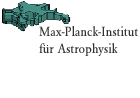General Relativistic Simulations of Rotational Supernova Core Collapse in Axisymmetry Quickfinder FAQ job vacancies   about the institute research groups local phone list weekly seminars preprints libraryMPA Homepage > Scientific Research > Research Groups > Relativistic Hydrodynamics EPS Figures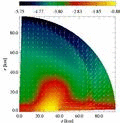H. Dimmelmeier
J.A. Font (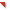Departamento de Astronomía y Astrofísica,Universidad de Valencia, Spain)
E. Müller

Figures of the waveforms in EPS format:

These figures contain the time evolution of the maximum density and the signal amplitude for all 26 simulated models. The solid and dashed lines denote the simulations in relativistic and Newtonian gravity, respectively.

The signal amplitude AE220 can be converted to the strain hTT in the equatorial plane at a distance r from the source by the following expression:

hTT = 1/8 sqrt(15/pi) AE220 / r = 8.8524 . 10-21 (AE220 / 1000 cm) (10 kpc / r).

The vertical dotted lines mark the time of peak maximum density (time of bounce), and the horizontal dotted lines in the density plots mark nuclear matter density, which we define as 2.0 . 1014 g/cm3.

For non-toroidal models, the maximum density is identical to the central density.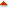Comments to: Harald Dimmelmeier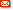harrydee@mpa-garching.mpg.de